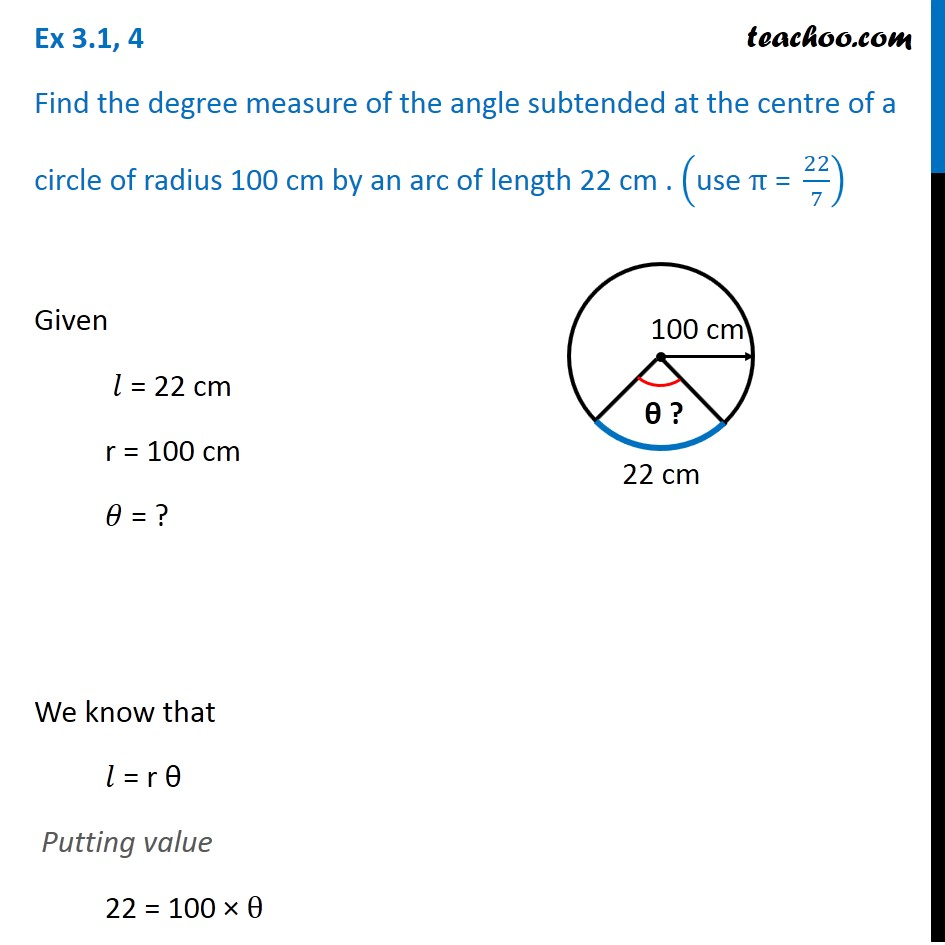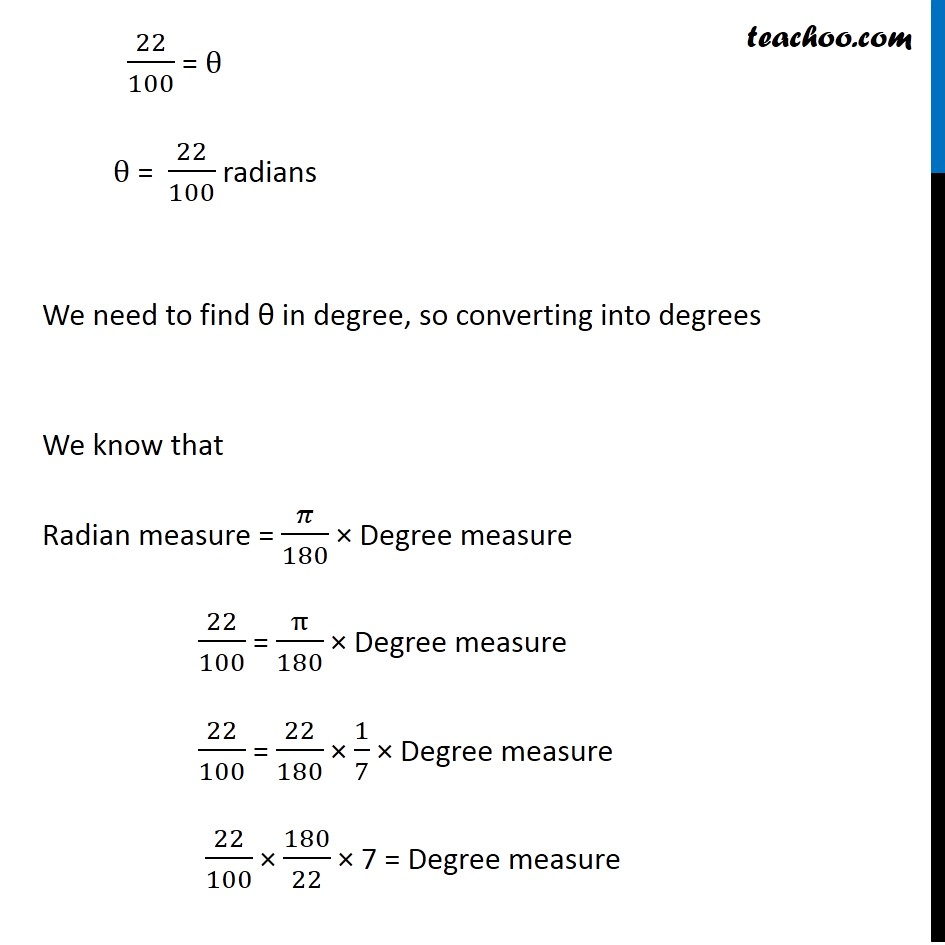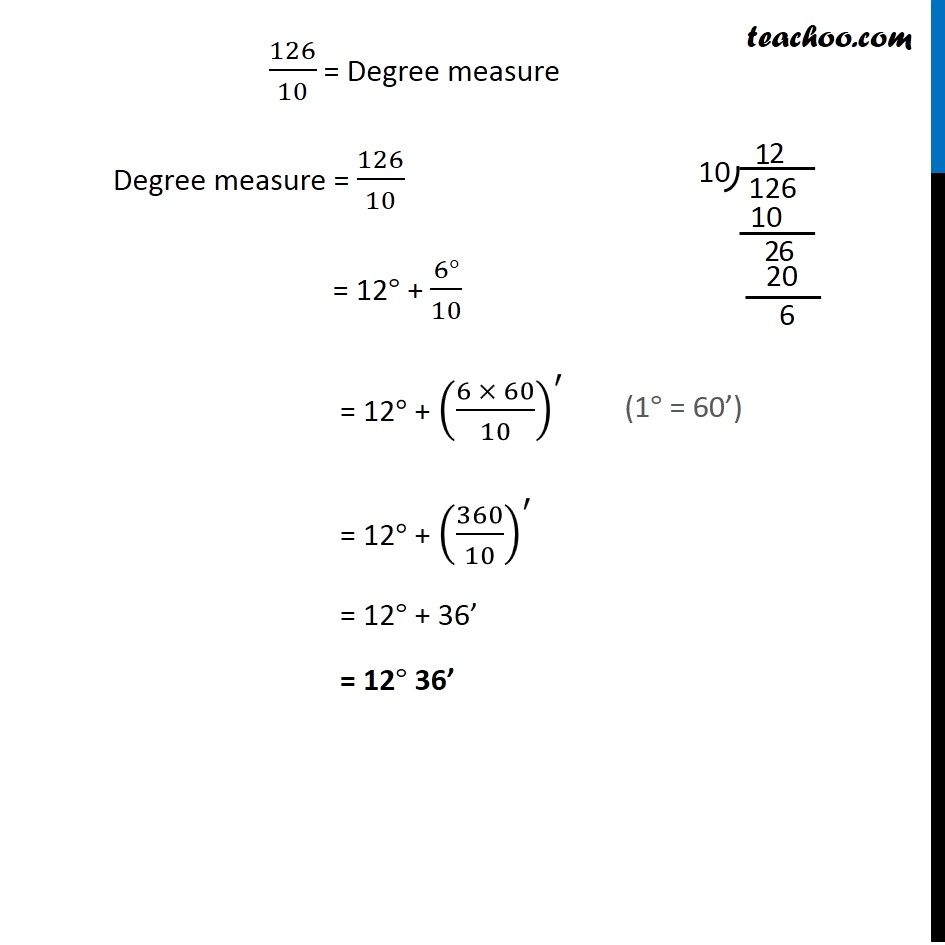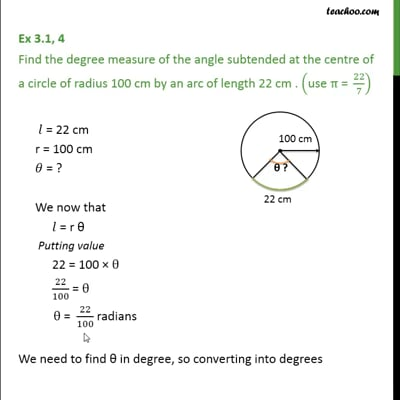Arc length

Chapter 3 Class 11 Trigonometric Functions
Concept wiseThis video is only available for Teachoo black users

Introducing your new favourite teacher - Teachoo Black, at only ₹83 per month

### Transcript

Ex 3.1, 4 Find the degree measure of the angle subtended at the centre of a circle of radius 100 cm by an arc of length 22 cm . ("use " π" = " 22/7) Given 𝑙 = 22 cm r = 100 cm 𝜃 = ? We know that 𝑙 = r θ Putting value 22 = 100 × θ 22/100 = θ θ = 22/100 radians We need to find θ in degree, so converting into degrees We know that Radian measure = 𝜋/180 × Degree measure 22/100 = π/180 × Degree measure 22/100 = 22/180 × 1/7 × Degree measure 22/100 × 180/22 × 7 = Degree measure 126/10 = Degree measure Degree measure = 126/10 = 12° + (6°)/10 = 12° + ((6 × 60)/10)^′ = 12° + (360/10)^′ = 12° + 36’ = 12° 36’